Rating5.00 / 5 Free EduCalc Classic

# EduCalc Classic

## Description

A powerful, flexible graphing calculator

Does far more than most calculators out there.

Features:

1) Scientific Calculator. Simple to grasp and easy to use, but powerful features are available when you need them. Available functions include the following:

• the usual arithmetic functions and exponentiation.
• square root, cube root, nth root, natural log, log base 10, log of arbitrary base, absolute value, factorial, permutations (nPr), combinations (nCr), modulus, random integer, bell curve, cumulative normal distribution, decimal to fraction.

2) Graphing. Capabilities:

• Graph up to four equations at once.
• Graphs are labeled.
• You can drag the graph or pinch to zoom in or out.
• Calculator can find roots and intersections.
• Graph in polar coordinates.
• Graph parametric equations
• Can graph implicit functions, such as x^2+y^2-4=0. Most calculator apps can’t do this!

3) A unit converter. With a tap, you can enter the result of your conversion into the calculator. Currently converts different units of the following: acceleration, angle, area, density, distance, energy, force, mass, power, pressure, speed, temperature, time, and volume. Great for doing physics homework!

4) Constants for scientific calculations — speed of light, strength of gravity at Earth’s surface, etc. etc. etc. Tapping on a constant will insert it into your calculation — i.e, you don’t have to key in the value. Again, great for doing physics homework!

5) It can make a table of the values of any function you care to enter. You can choose the starting x value of the table, as well as how much x increases for each successive row.

6) Help screens linked directly to many of the available functions and constants. Tap the disclosure arrow to see the definition.

7) Forgot the quadratic formula? Or the double-angle formulas for sine and cosine? The math/science reference hits the high points of various subjects. Currently includes algebra, differential and integral calculus, geometry, trigonometry, vectors, vector calculus, and classical mechanics.

8) Keep track of significant figures [AKA sig figs]

9) Statistics — enter data and make a histogram, box and whisker plot, or scatter plot with optional regression line.

If you are viewing this in iTunes, you will see five iPhone screenshots and five iPad screenshots. But even ten shots don’t come close to showing everything this calculator can do.

I’d love to hear your comments or suggestions. You can write me at mathscixyzgraphcalc@gmail.com — but without the xyz. Thanks!

## Screenshots

•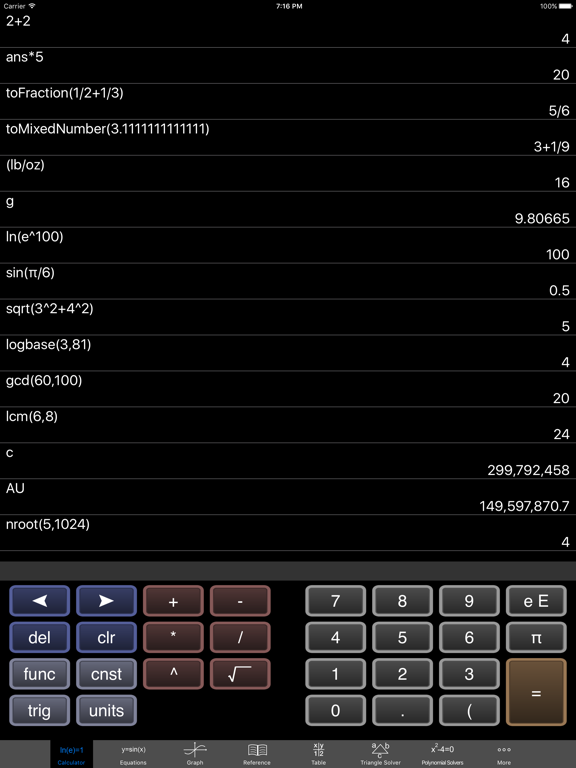•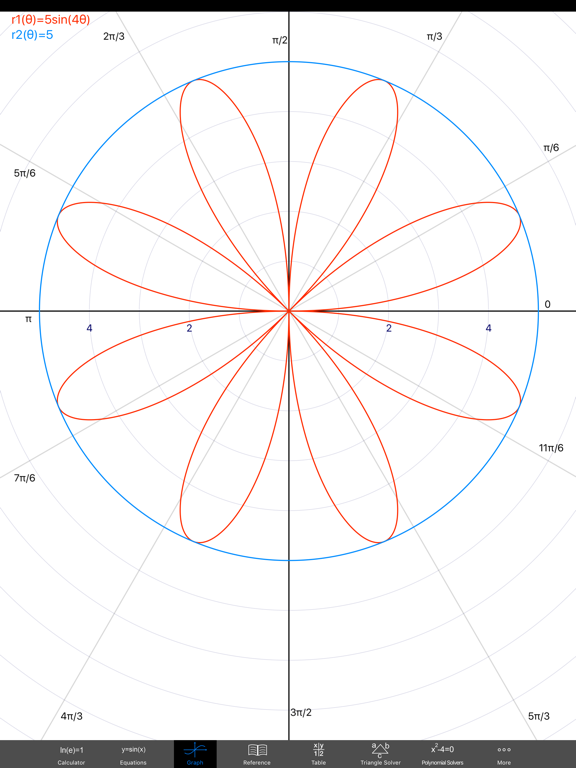•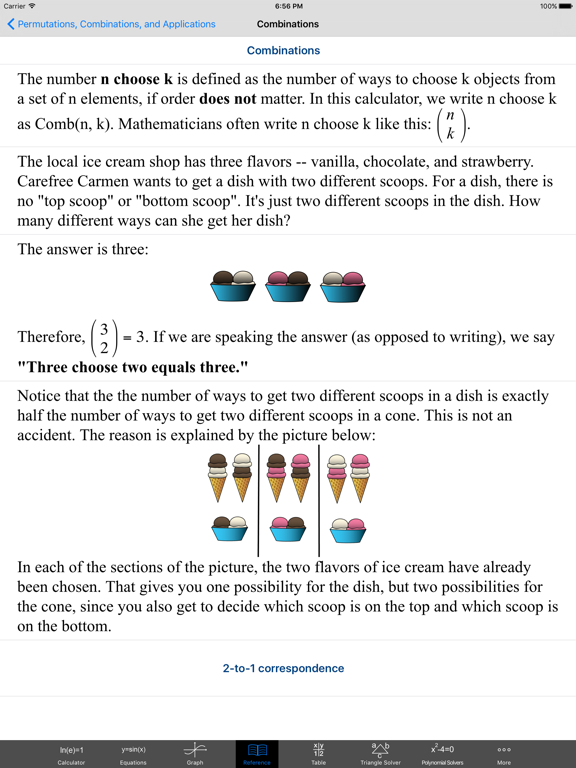•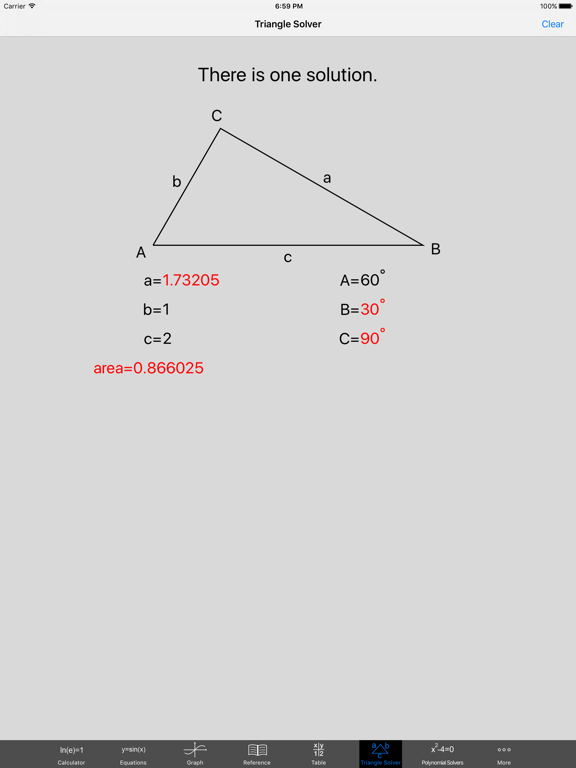•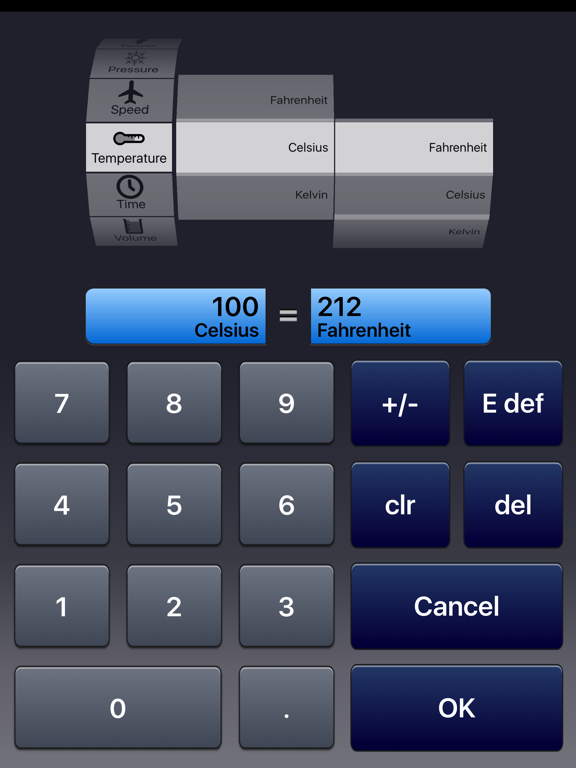## One Activity

1.Jennifer Caldwell - MCC says:

I chose this app because it allows students to explore the dynamics of linear equations on a deeper level than is allowed with traditional paper and pencil work. Students make generalizations and predictions about the effects varying values of slope. It also helped students to understand that the solution of a system of equations is the one ordered pair that the two lines have in common. Students were engaged when using this app.